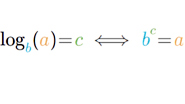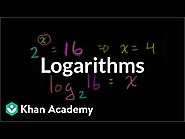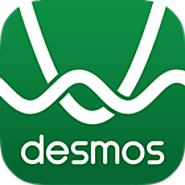Updated by Caleb Burnham on Apr 21, 2019
REPORTCaleb Burnham
Owner
10 items   1 followers   0 votes   2 views

# Curated Lesson Resources - Logarithms

1

## Khan Academy Intro to LogarithmsRationale: I chose this article because it has good explanations including color-coded equations. It also includes practice problems so students can chaeck their understanding

2

## Khan Academy Intro to logarithms VideoDescription: This video explains what logarithms are and gives a few examples of finding logarithms.
Rationale: I chose this as an alternative for visual learners to use to learn the material.

3

## Wolfram|Alpha: Making the world’s knowledge computableDescription: WoframAlpha is a computational search engine (fancy calculator) that students can use to find solutions to logarithms tied to their graphs.
Rationale: I chose this tool because it is a powerful; aid in doing homework and it will provide explanations of its solutions.

4

## DesmosDescription: Online graphing tool
Rationale: I included this tool because students can use it to easily create a graph using just an equation

5

## Graphing Calculator - GeoGebraDescription: Interactive, free online graphing calculator from GeoGebra.
Rationale: GeoGebra has a few more advanced features that Desmos does not, so I included it as an alternative if students want to get into some of the more complex manipulations of their graphs.

6

## PhotomathDescription: Photomath is a free mobile app that acts as an advanced graphing calculator that can scan equations from a page, even handwritten text!
Rationale: I included this resource because I know Siri can solve any high school math problem, so if my students are going to be using their phones to solve math problems I want them to have a tool that is easy to use and will give them the steps to solve the problems so they can understand what is going on.

7

## Introduction to Logarithms - Math Is FunDescription: An explanation of logarithms using bright colors and numerous examples.
Rationale: I chose this resource so that students can see more examples of logarithms if they need to and so they can get additional explanation if needed.

8

## Logarithm LawsDescription: An image with all the logarithm laws on it.
Rationale: I chose this resource so that my students can save this resource to their devices and use it for quick reference when working on an assignment.

9

## Uses of LogarithmsDescription: Explains what logarithms are used for and has some interactive elements to illustrate them.
Rationale: I chose this resource so that my students can understand why they are learning to use logarithms.

10

## Tricks to Help With Logarithms